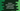# Python dictionary get() method explanation with example## How to use the get() method of python dictionary:

In python dictionary, we have one method called get that can be used to get the value of a key by using the key as the parameter. We can pass the key to the get method and it returns the value for that key.

Another way to get the value for a key is by using a square bracket. But there are differences between the square bracket and get. In this post, I will show you why you should prefer get and its advantange over the traditional way.

We will learn how to use get method and its advantage over square bracket.

### How and why to use get():

get takes the key of the dictionary as the parameter and returns the value for that key.

Let’s take a look at the below example:

``````given_dict = {'one': 1, 'two': 2, 'three': 3}

print(given_dict['one'])
print(given_dict['four'])``````

Here,

• given_dict is a dictionary with three key-value pairs.
• The first print statement is printing the value for key one.
• The second print statement is printing the value for key four. But, we don’t have any key four. So, it will throw one error.
``KeyError: 'four'``

It will print the first value and throw KeyError for the second one.

Now, if we use get, it looks as like below:

``````given_dict = {'one': 1, 'two': 2, 'three': 3}

print(given_dict.get('one'))
print(given_dict.get('four'))``````

It will not throw any error, but print None for the second statement.

``````1
None``````

This is the benifit of using get.

We can also optionally pass the value that needs to be returned if the key is not found. This value needs to be passed on the second parameter.

``````given_dict = {'one': 1, 'two': 2, 'three': 3}

print(given_dict.get('one'))
``````1